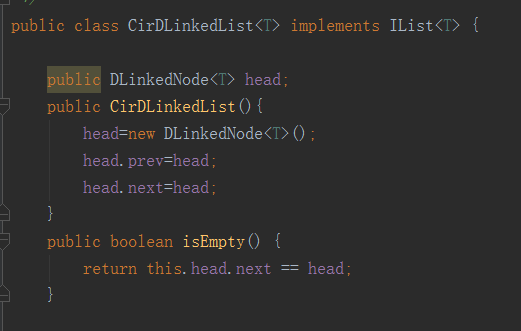# Java数据结构之线性表

更新时间：2017年03月10日 10:19:19   转载 作者：朝向远方

1：线性表抽象的数据类型

```public interface IList<T> {
/**
* 是否为空
* @return
*/
boolean isEmpty();
/**
* 表的长度
* @return
*/
int length();
/**
* 根据索引获取长度
* @param i
* @return
*/
T get(int i);
/**
* 设置第i个元素值为x
* @param i
* @param x
*/
void set(int i,T x);
/**
* 在线性表最后插入x元素
* @param x
*/
void append(T x);
/**
* 异常第i个元素并返回值
* @param i
* @return
*/
T remove(int i);
/**
* 删除线性表中所有元素
*/
void removeAll();
/**
* 查询首次出现关键字为key的元素
* @param key
* @return
*/
T search(T key);
void insert(int i,T x);
/**
* 升序添加
* @param x
*/
void insert(T x);
/**
* 升序删除
* @param x
*/
void remove(T x);
}```

2：线性表顺序表示和实现3：顺序表操作效率分析

4：单链表的实现

```public class Node<T> {
public T data;//数据域
public Node next;//地址域
public Node() {
this(null, null);
}
public Node(T data, Node next) {
this.data = data;
this.next = next;
}
}
```

```Node<String> A=new Node<T>(x,null);
Node<String> B=new Node<T>(y,null);
A.next=B。
```

ok现在我们正式开始实现一个带有头节点的单链表

```public int length() {
int i = 0;
while (p != null) {
p = p.next;
i++;
}
return i;
}```

```public T get(int i) {
if (i >= 0) {
for (int j = 0; j <= i; j++) {
p = p.next;
}
if (p!=null){
return p.data;
}
}
return null;
}```insert(Integer.MAX_VALUE, x)即可，从插入代码可以看到当循环到最后一个节点就不会继续了，然后把新的节点加入最后即可。

5：排序单链表6：单链表操作效率分析

7：双链表的实现8：循环双链表